## Geometry

Theory

One of the hardest sections to crack without preparation and one of the easiest with preparation. With so many formulas to learn and remember, this section is going to take a lot of time to master. Remember, read a formula, try to visualize the formula and solve as many questions related to the formula as you can. Knowing a formula and knowing when to apply it are two different abilities. The first will come through reading the formulae list and theory but the latter can come only through solving many different problems.

Formula

Basic Formulae: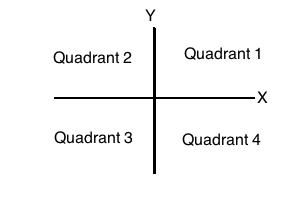• The distance between two points with coordinates $$(x_1, y_1), (x_2, y_2)$$ is given by $$d = \sqrt{(x_2-x_1)^2+(y_2-y_1)^2}$$
• A line can be defined as $$y=mx+c$$ where m is the slope of the line and c is the y-intercept
• When two lines are parallel, their slopes are equal i.e $$m_1=m_2$$
• When two lines are perpendicular, product of their slopes = -1 i.e $$m_1*m_2=-1$$
• If a and b are the x and y intercept of a line then x/a+y/b=1
Tip
• When two angles A and B are complementary, sum of A and B=90 degrees
• When two angles A and B are supplementary, sum of A and B=180 degrees
• When two lines intersect, opposite angles are equal. Adjacent angles are supplementary
• When any number of lines intersect at a point, the sum of all the angles formed = 360 degrees
Theory

Consider parallel lines AB, CD and EF as shown in the figure.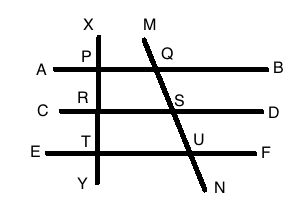• XY and MN are known as transversals

• $$\angle$$ XPQ = $$\angle$$ PRS = $$\angle$$ RTU as corresponding angles are equal

• Interior angles on the side of the transversal are supplementary. i.e. $$\angle$$ PQS + $$\angle$$ QSR = 180 degrees

• Exterior angles on the same side of the transversal are supplementary. i.e. $$\angle$$ MQB + $$\angle$$ DSU = 180 degrees.

• Two transversals are cut by three parallel lines in the same ratio i.e. PR / RT = QS / SU

Theory

### Triangles

• Sum of all angles in a triangle is 180 degrees
• An angle less than 90 degree is called an acute angle. An angle greater than 90 degrees is called an obtuse angle.

Nomenclature

• A line joining the mid point of a side with the opposite vertex is called a median.* A perpendicular drawn from a vertex to the opposite side is called the altitude

• The three perpendicular bisectors of a triangle meet at a point called the Circumcentre. A circle drawn from this point with the circumradius would pass through all the vertices of the triangle.
• The three angle bisectors of a triangle meet at a point called the incentre of a triangle. The incentre is equidistant from the three sides and a circle drawn from this point with the inradius would touch all the sides of the triangle.
• The point of intersection of the three altitudes is the Orthocentre
• The point of intersection of the three medians is the centroid

• A triangle with two sides equal is called an isosceles triangle. A triangle with all sides equal is called an equilateral triangle. The two angles of an isosceles triangle that are not contained between the equal sides are equal. All angles of an equilateral triangle equal 60 degrees.

• Sum of any two sides of a triangle is always greater than the third side
• Pythogoras theorem: In a right angled triangle ABC where $$\angle$$B=90 degrees, $$AC^2$$ = $$AB^2$$ + $$BC^2$$
Tip

Important Points to Remember:

• All points on an angle bisector are equidistant from both arms of the angle.
• All points on a perpendicular bisector of a line are equidistant from both ends of the line.
• In an equilateral triangle, the perpendicular bisector, median, angle bisector and altitude drawn from a vertex to a side coincide.
• If x is the side of an equilateral triangle the altitude is $$\frac{\sqrt{3}}{2}x$$.
• In a triangle ABC if AD is the angle bisector of angle A then AD bisects the side BC in the same ratio as the other two sides of the triangle. i.e. BD/ CD= AB/AC.
Theory
• Consider the triangle ABC with incentre I, and the incircle touching the triangle at P,Q,R as shown in the diagram. As tangents drawn from a point are equal, AP=AQ, CP=CR and BQ=BR.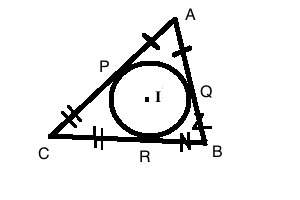• In an equilateral triangle, the centroid divides the median in the ratio 2:1. As the median is also the perpendicular bisector, angle bisector, G is also the circumcentre and incentre.
• If a is the side of an equilateral triangle, circumradius = $$a/\sqrt{3}$$ and inradius = $$= a/2\sqrt{3}$$.
Theory
• Consider a circle as shown in the image. Here, $$AP$$ * $$AQ$$ = $$AS$$ * $$AU$$ = $$AT^2$$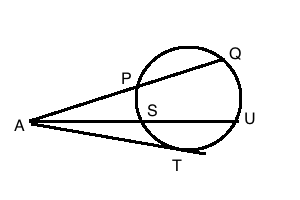• Two chords that are equal in length will be equidistant from the centre and vice versa
• When two circles intersect the line joining the centres perpendicularly bisects the line joining the points of intersection
• The angle made by a chord with a tangent to one of the ends of the chord is equal to the angle subtended by the chord in the other segment. As shown in the figure, angle ACB = angle BAT.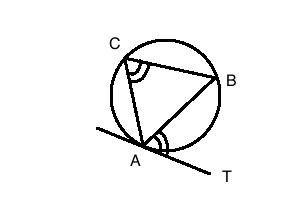Theory

Circle Properties

• The angle subtended by a diameter of circle on the circle = 90 degrees
• Angles subtended by a chord are equal. Also, angles subtended in the major segment are half the angle formed by the chord at the centre
• The radius from the centre to the point where a tangent touches a circle is perpendicular to the tangent
• Tangents drawn from the same point to a circle are equal in length
• A perpendicular drawn from the centre to any chord, bisects the chord
• A polygon of n sides has n(n-3)/2 diagonals
• In a regular polygon of n sides, each exterior angle is 360/n degrees.
Formula

Angle subtended by a chord on the circumference is half of the angle subtended by the same chord on the centre.

Formula

Surface area of different solids:

• Prism SA= 2* base area+ base perimeter*height
• Cube $$SA$$ =  $$6$$ * $$length^2$$
• Cylinder $$SA$$ = 2 $$\pi rh$$ + 2 $$\pi r^2$$
• Pyramid SA = 1/2 *Perimeter of base *slant height+Area of base* Cone $$SA$$ = $$\pi r (l+r)$$ where r is radius of base and l is the slant height
• Cone frustum $$SA$$ = $$\pi(R^2+r^2+R*l+r*l)$$ where R and r are the radii of the base faces and l is the slant height
Formula

Area of a triangle A

• $$A$$ = $$\sqrt{s(s-a)(s-b)(s-c)}$$ where s = (a+b+c)/2.
• A = 1/2 * base * altitude
• A = 1/2 ab sinC
• A = abc/4R where R is the circumradius
• A = rs where r is the inradius and s is the semiperimeter
Formula

• For a cyclic quadrilateral, $$A$$ = $$\sqrt{(s-a)(s-b)(s-c)(s-d)}$$ where s=(a+b+c+d)/2
• A = 1/2 * One diagonal * Sum of offsets drawn to the diagonal
• For a trapezium, Area = 1/2 * sum of parallel sides * distance between them
• For a parallelogram, A=Base * Height = Product of two sides * sine of included angle
• For a rhombus = 1/2 * Product of diagonals
• For any solid, Euler's rule states that: Number of faces + Number of vertices = Number of edges +2
Formula

Volume of different solids:

• Cube $$V$$ = $$length^3$$
• Cuboid V = length* base*height
• Prism V = Area of base * height
• Cylinder $$V$$ = $$\pi r^2 h$$
• Pyramid V=1/3 *Area of base * Height
• Cone $$V$$ = 1/3 * $$\pi r^2 *h$$
• Cone Frustum where R is the base radius, r is the upper surface radius and h is the height of the frustum $$V$$ = 1/3 $$\pi h (R^2+Rr+r^2)$$
Formula

Apollonius theorem states that in a triangle ABC, if AD is a median to BC then $$AB^2+AC^2=2\cdot\left(AD^2+BD^2\right)$$

Theory

Apollonius theorem

In a triangle ABC, if AD is the median to side BC then by Apollonius theorem, $$2*(AD^2+BD^2)$$ = $$AC^2$$ + $$AB^2$$.